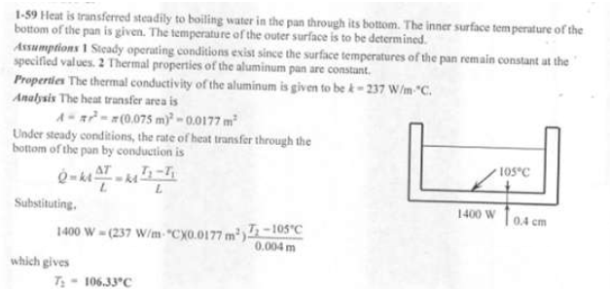heat and mass transfer problems engineering equations heat and mass transfer school homework engineering heat and mass transfer formulas heat and mass transfer solutions to heat and mass transfer problems full solution engineering problem solution heat and mass transfer math problems engineering equations heat and mass transfer school homework engineering solutions to heat and mass transfer formulas heat problems mass problem solutions to transfer problems full solution heat and mass transfer
heat and mass transfer problems engineering equations heat and mass transfer school homework engineering heat and mass transfer formulas heat and mass transfer solutions to heat and mass transfer problems full solution engineering problem solution heat and mass transfer math problems engineering equations heat and mass transfer school homework engineering solutions to heat and mass transfer formulas heat problems mass problem solutions to transfer problems full solution heat and mass transfer
Highalphabet Home Page heat and mass transfer problem solutions Heat and Mass Transfer Page
An aluminum pan whose thermal conductivity is 237 W/mK has a flat bottom with diameter 15 cm and thickness 0.4 cm. Heat is transferred steadily to boiling water in the pan through its bottom at a rate of 1400 W. If the inner surface of the bottom of the pan is at 105 C, determine the temperature of the outer surface of the bottom of the pan.An aluminum pan whose thermal conductivity is 237 W/mK has a flat bottom with diameter 15 cm and thickness 0.4 cm. Heat is transferred steadily to boiling water in the pan through its bottom at a rate of 1400 W. If the inner surface of the bottom of the pan is at 105 C, determine the temperature of the outer surface of the bottom of the pan.